Get instant live expert help with Excel or Google Sheets“My Excelchat expert helped me in less than 20 minutes, saving me what would have been 5 hours of work!”

#### Post your problem and you’ll get expert help in seconds.

Your message must be at least 40 characters
Our professional experts are available now. Your privacy is guaranteed.

# How to Sum Values in Excel If They Are Not Blank

We can sum cells when certain values are not blank. We can do this with the SUMIF function. This step by step tutorial will guide all levels of Excel users through summing all non-blank values.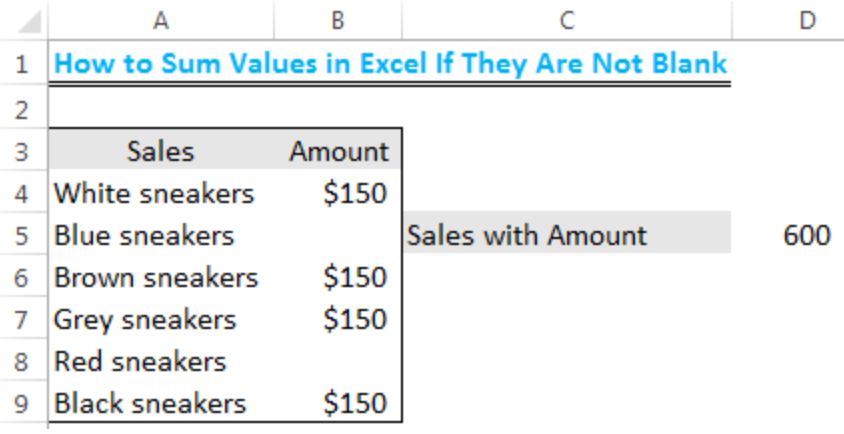Figure 1: Result of Summed Values that are not blank

## Setting up the Data

• We will set up our data by inputting the SALES and AMOUNT in Column A and Column B respectively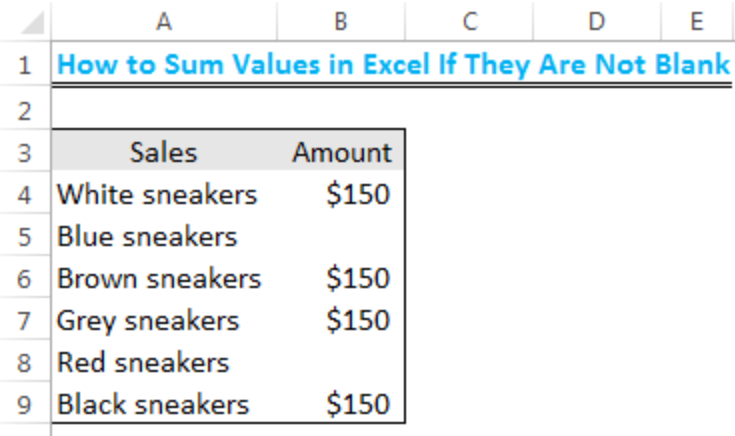Figure 2: Setting up the Data

## Formula

`=SUMIF(A4:A9,"<>",B4:B9)`

## Syntax

`=SUMIF(range,"<>",sum_range)`

## Explanation

With this formula, we will sum the amounts in Column B provided the corresponding Cell in Column A is not blank.

The criteria “<>” is used to indicate cells that are not empty.

## Summing the Values

• We will name Cell C5 as Sales with Amount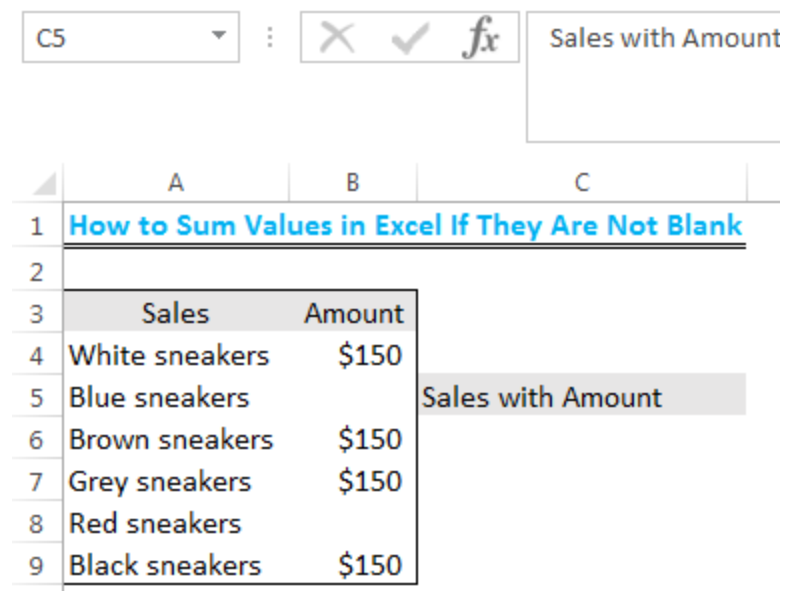Figure 3: Summing Values that are not blank

• We will input the formula into Cell D5; `=SUMIF(A4:A9,"<>",B4:B9)`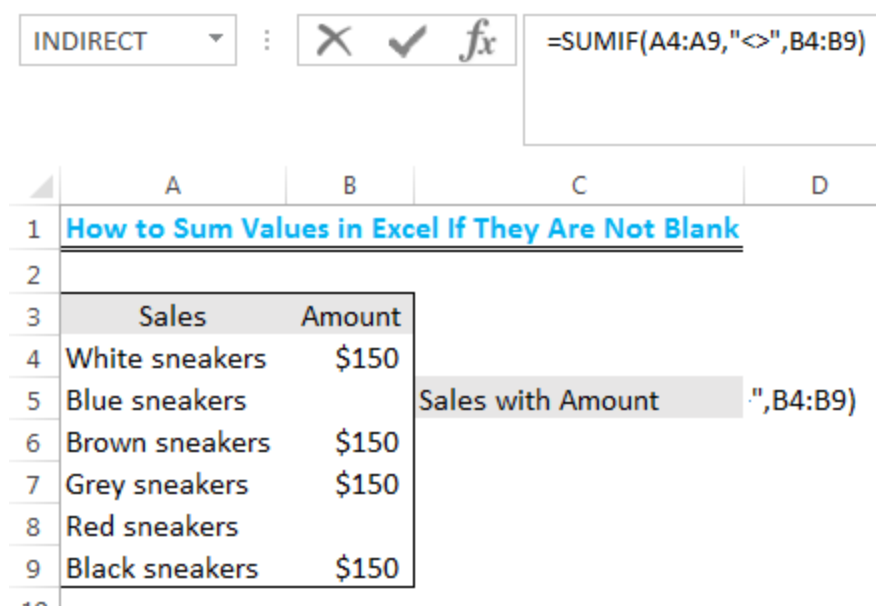Figure 4: Summing Values that are not blank

• Now, we will press ENTER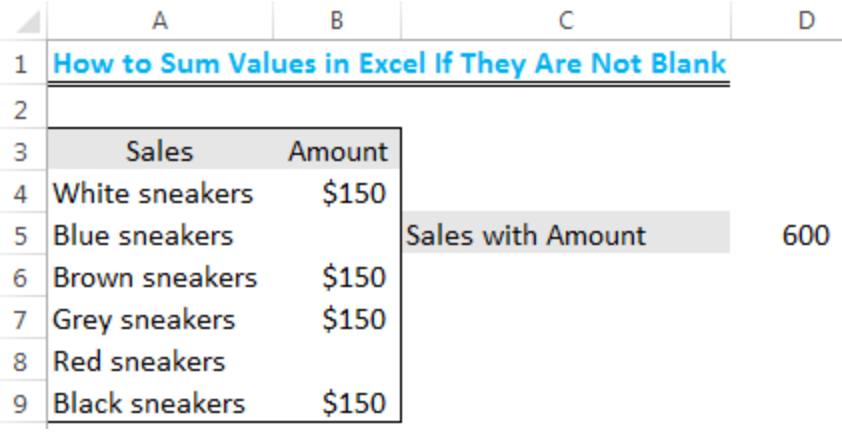Figure 5: Result of Summed Values that are not blank

## Instant Connection to an Expert through our Excelchat Service

Most of the time, the problem you will need to solve will be more complex than a simple application of a formula or function. If you want to save hours of research and frustration, try our live Excelchat service! Our Excel Experts are available 24/7 to answer any Excel question you may have. We guarantee a connection within 30 seconds and a customized solution within 20 minutes.

Solution examplesamending a formula so that there is another criteria and if it does not meet this criteria then we stick with the original criteria
Solved by A. B. in 40 minsI need Column D to = Column C - Column A only if Column B has a 'Date' entered.
Solved by I. B. in 11 minsHello, I need help trying to create a sum of numerical values on a spreadsheet in which the only values that apply to the sum are when the status of another cell is a certain value (e.g. value is not in sum when status: closed, value is in sum when status: open)
Solved by T. W. in 18 minsI am looking for a formula to SUM column L, if Column A matched the relevant date and column C matches the name
Solved by Z. Y. in 16 minsI need a formula that is essentially saying IF this range of cells contain a certain name, add the number in another cell on the same row, and them sum that number in a different cell..!
Solved by O. H. in 44 mins